## Problem statement

If we list all the natural numbers below 10 that are multiples of 3 or 5, we get 3, 5, 6 and 9. The sum of these multiples is 23.

Find the sum of all the multiples of 3 or 5 below 1000.

## Thoughts

This can easily be solved without any loops.

## Solution

As we want values below 1000, we use limit 999

int limit = 999;


There exists the following equation for summing natural numbers up to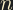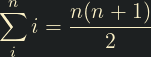int getSumNats(int limit)
{
return limit * (limit + 1) / 2;
}


Consider the following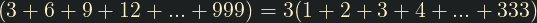We can recognise, therefore, that we can determine the sum of multiples of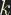by setting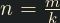, and multiplying by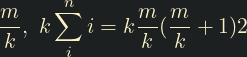So to calculate the sum of the multiples of 3 and 5 under 1000 we need to:

• Add the sum of the multiples of 3

• Add the sum of the multiples of 5

• Subtract the sum of the multiples of 15 (to avoid counting them twice)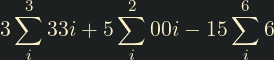#include <stdio.h>
int main()
{
int sum_multiples_of_3 = 3 * getSumNats(limit / 3);
int sum_multiples_of_5 = 5 * getSumNats(limit / 5);
int sum_multiples_of_15 = 15 * getSumNats(limit / 15);

printf("%d\n",
sum_multiples_of_3 + sum_multiples_of_5 - sum_multiples_of_15);
}